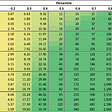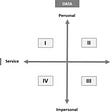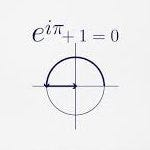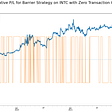# The Realized Volatility Puzzle

Back when I was interviewed for my first job in finance I got a pretty simple assignment (or at least that’s what I thought…). I was given an historical data set of EUR/USD returns (daily closing price/High/Low) and I was supposed to make a decision whether to buy a 1-month volatility based on that.

My first impression was “ok, that’s an easy one, let’s just calculate the historical 1-month close-close volatility and compare that to the implied, and so I did”. At the interview the head of the Quant Research team asked me “what made you use 1-month realized close-close volatility, and not high/low volatility. Also, why did you assume that looking back at the 1-month volatility is a good gauge to make decision with regards to the implied?”

luckily this was a junior quant job, so the bar for accomplishing the assignment was pretty low, and I got the job, but that made me so intrigued about the, rather trivial, measurement of historical volatility.

Let’s first acknowledge what realized volatility is NOT — it is not a perfect predictor to future volatility (otherwise the entire complex of volatility trading would have been obsolete). Many academic papers and works have tried forecasting volatility using Autoregressive models (ARMA , GARCH), and while these models work on the theoretical level, there is little to no use for them in practice (I’ve seen very few practitioners using them as their main tool in option modeling/trading).

So, if we can’t use it to forecast future realized volatility, why should we care about measuring historical volatility?

As practitioners in financial markets, we often have nothing but historical data sets to make trading/strategy decisions (is volatility too rich/cheap, what volatility regime we are in at the moment, how volatile has stock xyz been lately..). Obviously, given that our most powerful tool in quantitative trading is the ability to analyze (and manipulate) data sets, we should have deep understanding about the measurement of realized volatility.

Let’s dive into the realized volatility measurement, and the different factors affecting it.

Length and Frequency of realized volatility:

The selection of length/frequency is probably the first and foremost important factor of the realized volatility measurement. While we sometimes put little to no thought into the realized volatility length/frequency, this is far from being a trivial question. Let’s say that we want to analyze the 1-month volatility. Which lookback window should we look at? should we compare that to the realized 10-day/20-day/40-day or even 100-day? what can assure us that the recent 20-day volatility is going to be a suitable comparison for the future 1-month?. Furthermore, how frequent should we sample the data? should we sample returns on close-close basis, or should we sample the data using hourly/tick data?

Let’s look at a sample plot of USDJPY o/n realized vol against the previous day realized vol (or in other words, auto-regression)

If we look at the o/n realized volatility of USDJPY regressed against a lagging 1-day value, we see that there is a degree of predictability, however, what happens if we try to do the same for longer tenors (i.e. longer tail)?

If we take USDJPY 2-weeks realized vol against 2-week lagged value we see lesser degree of predictability:

and we can assume that the longer our tail (i.e. the tenor that we are trying to predict or estimate), the less useful it will be to use realized volatility as a predictor.

When it comes to the sampling frequency it boils down to the length of realized volatility tenor. If we look at USDJPY as a test case, we can see a decreasing difference between Daily (1 sample/day) and High-Frequency sampling (48 samples/day).

These results are rather intuitive, as short term realized vol has less data points which makes the weight of each data point greater on the overall variance (and therefore, realized vol), so as our realized volatility look period increases the less meaningful the sampling frequency.

# One Realized Vol , Four different ways to measure…

Up until now we only discussed the length and sampling frequency, under the assumption that there is only one way to measure realized volatility — as a function of fixed T (time) intervals, however there are actually (at least) four different ways to measure realized volatility:

1. Close-Close basis :

The common (and familiar) way to measure realized volatility — as function of fixed T (time) interval. A c-c (close-close) realized volatility can be of any time interval we want (it can be daily intervals, hourly, half-hourly, and even nano-seconds).

This measuring method is widely used as it’s a pretty straightforward calculation, however, it lacks to take into account few dynamics that tend to happen in financial markets. The two most notable assumptions of this measurement are :

1. Return are i.i.d (zero autocorrelation between returns),

2. We trade in discrete time (i.e., fixed time intervals).

While we can live with the first assumption, the 2nd assumption is just inaccurate for most times. We rarely trade in discrete times (just think about a trader saying “I will trade ONLY at the close/open (or any other fixed time interval)”… The only traders i know that doing that are exotic traders who hedge VarSwap/VolSwaps (or any other fixing based products).

2. Parkinson Volatility — Unlike close-close volatility, Parkinson Volatility uses high/low price of the underlying at a given sample.

While this makes Parkinson better estimator than close-close, as it’s less sensitive to sample intervals , it doesn’t account for jumps (intraday/close-open/weekends)

3. Garman Klass Volatility — As we saw, Parkinson Volatility improves the estimation (as it accounts for intraday high/low and reduces the path dependency). That said, it fails to account for intraday price dynamic. Garman Klass Volatility does address the intraday dynamic by accounting for open/close price.

While this takes us one step further in improving the estimation of realized volatility, it does not address jumps, and tend to underestimates realized volatility

4. GKYZ Volatility — As we saw previously all realized volatility measures failed to address discontinuous price dynamic (which is extremely important in markets that are not trading 24h, like stocks and some commodities). Yang-Zhang volatility estimator (extension of Garman Klass) addresses most aspects of realized price dynamic and can handle intraday dynamic, as well as overnight jump, and is considered the most efficient estimator.

# Different uses of realized volatility

As we noted previously, the predictive power of realized volatility is somewhat limited, yet realized volatility is probably one of the most useable measurements we have in our quantitative arsenal when analyzing and trading volatility. Although there are many applications of realized volatility I will focus on three that I find very handy for volatility practitioners:

1. Analysis of Implied volatility relative richness/ cheapness — Although we acknowledge that realized volatility can tell us very little about the future, it uses as our base vol (using different look back periods) when pricing implied volatility. While implied volatility construction methods is a very deep topic (that I probably will address somewhere down the road in a dedicated post), I want to show a very rough methods used by dealers/market-maker to estimate the ATM implied volatility.

Let’s assume that we are looking to price 1-month SPX implied volatility and the information we have is SPX historical 1-month time series and the upcoming economic calendar. We can look at realized volatility of 24h/1w/2w/1m tenors and estimate the average realized volatility. Now, obviously if we price the implied volatility at the average realized volatility we assume that for the next 1-month the SPX will realize the average past 1-month(i.e. zero volatility risk premium) , but what if there are economic events (FOMC, NFP, GDP, etc..) that can make the SPX price dynamic more volatile- so we apply multipliers/factors on the realized volatility that we measured (using historical comparable events and realized price action), and weigh the “average o/n vol” and “event vol” . The end product is a kind of weighted average between the two.

2. Estimating Mean-Reversion/Trend dynamic (Variance Ratio) — As discussed in previous my previous post about Delta Hedging, We can use different realized volatility estimators to detect mean-reversion/trend dynamic. Variance Ratio test is a simple ratio of High Frequency realized variance measurement (Garman Klass for example) to Daily close-close realized variance. The idea behind this test is rather intuitive, as mean reverting dynamic will exhibit higher intraday variance, while low close-close variance. If we look at the SPX/ Variance Ratio plot below, we can see that the SPX chart tells us only part of the story (i.e., the long term dynamic), while the Variance Ratio gives us information about the short-term spot dynamic

3. Analyzing realized volatility dynamic under different regimes — One of the best applications of realized volatility (and timeseries analysis in general) is the ability to analyze volatility (and spot) dynamic under different market regimes. We can use past realized volatility to make assumptions with regards to future realized volatility dynamic if we believe that these regimes are going to exhibit similarities. Let’s consider the following example : We are considering buying 1–week ATM USDJPY volatility to trade the upcoming FOMC meeting (perhaps the market is split with regards to the forward guidance/possible QE4/balance sheet adjustment), and we want to estimate how much the market is likely to move over the week following the meeting. We can analyze past FOMC meeting (and following price actions) that exhibited the same features.. Obviously each dynamic can be unique, but it gives us a good approximation (in the absence of a magic 8 ball…)

# Ok, So what realized volatility measurement should we use?

As we know by now that there is not one “correct” way to measure realized volatility, we need to figure out which realized volatility measure compliments our needs to best. After years of trails and errors I came up with something I like to call “The practitioners guide to realized volatility”

The rule of thumb (as I see it) for volatility practitioners is as follows:

1. Role — risk managers, who run risk assessments (VaR and its kinds), are less likely to focus on tick-by-tick volatility, therefore more likely to use close-close (daily samples) volatility (and its derivatives, such as semi-variance/expected shortfall/etc…). On the other hand, traders, who run option books and dynamically hedging them are more sensitive to intraday volatility and price dynamic.
2. Asset Class- Not all assets classes are made equal. While Rates and FX (G10 mostly) exhibit less jumps (discontinuity), in Equity/ Emerging Markets/ Commodities market there is a higher likelihood to see discontinuity in price dynamic (and therefore, realized volatility measurement should accommodate these dynamics)
3. Product specifications — Some products, such as Variance/Volatility/Correlation swaps are fixed daily (using a specific fixing source/time). This specification makes them less sensitive to the intraday price dynamic, and more sensitive to the actual fixing. On the other hand, option books that are being managed dynamically (and have higher path dependency/gamma exposure), are more sensitive to intraday volatility dynamic, and therefore practitioners should assess the “True” volatility of the asset.

Obviously this is just the tip of the volatility iceberg… I believe that practitioners should fully understand volatility estimators inside-out, as these nuances sometimes will make the difference between a profitable trade and a losing trade.

Feel free to comment and share your thoughts…

--

--

--

## More from The Startup

Get smarter at building your thing. Follow to join The Startup’s +8 million monthly readers & +756K followers.

## A simple Covid19 API## Anomaly Detection with Multi Dimensional Time Series Data## GVT \$94k Part 5: Historical Price Movement and Modeling## GA COVID-19 Report September 24, 2021## Pytorch Training Tricks and Tips## Problems with Multiple Linear Regression, in R## Open data information community: library and information centre resources and services## An interview with Abhishek Kumar, Senior Manager, Data Science at Publicis Sapient## A Leading Fundamental Indicator for Equity Investing.## Think Like a Market Maker — Understanding Implied Volatility## Optimizing a Trading Strategy without Backtesting the Alpha## The Gamma Exposure Trading Strategy.